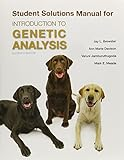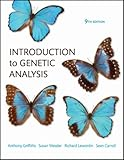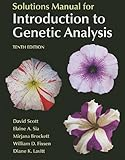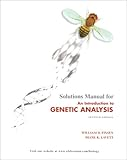Introduction to Genetic AnalysisSolutions Manual for Introduction to Genetic AnalysisIntroduction to Genetic AnalysisIntroduction to Genetic Analysis, 9th EditionIntroduction to Genetic Analysis, Solutions ManualIntroduction to Genetic AnalysisThe Cognitive Neurosciences (The MIT Press)Solution manual for An Introduction to Genetic AnalysisAn Introduction to Genetic Analysis & CD-RomPrinciples of Life (Loose Leaf) & Launchpad 24 Month Access Card